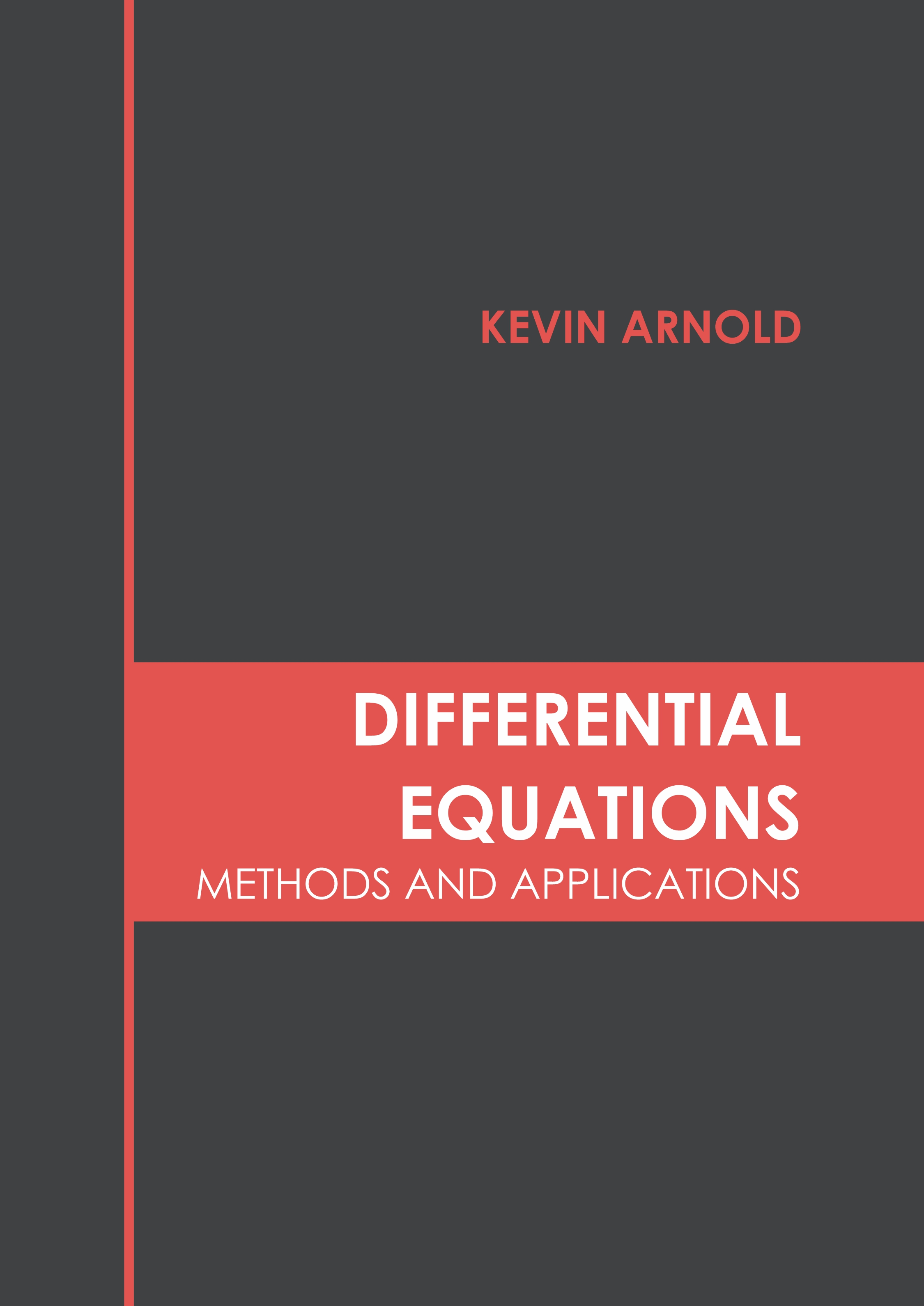• library@msu.ac.th
• Academic Resource Center Mahasarakham University
• library@msu.ac.th
• Academic Resource Center Mahasarakham Universityหมวดหมู่### Differential Equations: Methods and Applications

Mathematical equations which can be used to establish a relation between various functions and their derivatives are known as differential equations. They can be used for representing physical quantities, their rates of change, and a correlation between the two. Differential equations can be classified into ordinary differential equations, non-linear differential equations and partial differential equations. These can also be divided on the basis of their order. The order of a differential equation is determined by the term having the highest derivative. The various sub topics of this discipline are delay differential equations, integro-differential equations, stochastic differential equations, etc. The subject of differential equations finds extensive application in the fields of physics, engineering, chemistry, biology and economics. This book presents the complex subject of differential equations in the most comprehensible and easy to understand language. Different approaches, evaluations and methodologies on differential equations have been included herein. Those in search of information to further their knowledge will be greatly assisted by this book.

ใส่ตะกร้า
• ISBN9781639891535
• ประเภท E-Book
• ผู้แต่ง Kevin Arnold